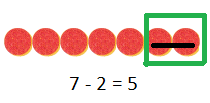# Texas Go Math Grade 1 Lesson 11.3 Answer Key Subtract to Compare

Refer to our Texas Go Math Grade 1 Answer Key Pdf to score good marks in the exams. Test yourself by practicing the problems from Texas Go Math Grade 1 Lesson 11.3 Answer Key Subtract to Compare.

## Texas Go Math Grade 1 Lesson 11.3 Answer Key Subtract to Compare

Explore

Useto show the problem. Draw the. Model the problem using the model.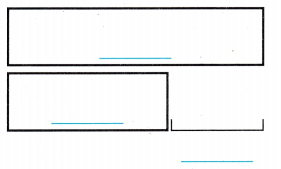FOR THE TEACHER • Read the problem. Mindy I has 8 puzzle pieces. David has 5 puzzle pieces. How many more puzzle pieces does Mindy have than David?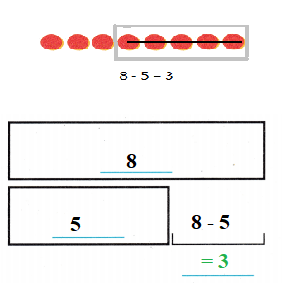Explanation:
Given,
Mindy has eight puzzles pieces,
David has five puzzle pieces,
By subtracting five from eight we get three,
So Mindy has three more puzzle pieces than David.

Math Talk
Mathematical Processes

Explain how you find how many more puzzle pieces Mindy has than David.
Answer:  Eight minus five is equal  to ThreeBy subtracting method, we can get the count of puzzle pieces that Mindy has more than David.
By subtracting five from eight we get three,
So Mindy has three more puzzle pieces than David.

Model and Draw

James has 4 stones. Heather has 7 stones. How many fewer stones does James have than Heather?__________ fewer stones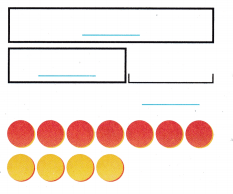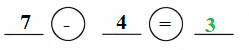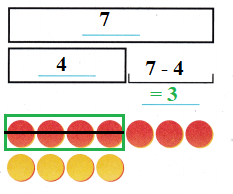Explanation:
Given,
James has four stones,
Heather has seven stones,
By subtracting four from seven we get three,
So James has three fewer stones than Heather.

Share and Show

Useto show the problem. Use the model to solve. Write the number sentence. Then write how many.

Question 1.
Abby has 8 stamps. Ben has 6 stamps. How many more stamps does Abby have than Ben?
_______ more stampsExplanation:
Given,
Abby has eight stamps,
Ben has six stamps,
By subtracting six from eight we get two,
So Abby has two more stamps than Ben.

Question 2.
Tanner has 3 books. Vicky has 6 books. How many fewer books does Tanner have than Vicky?
__________ fewer books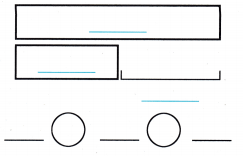Explanation:
Given,
Tanner has three books,
Vicky has six books,
By subtracting three from six we get three,
So Tanner has three fewer books than Vicky.

Problem Solving

Useto show the problem. Use the model to solve. Write the number sentence. Then write how many.

Question 3.
Sally has 5 feathers. James has 2 feathers. How many more feathers does Sally have than James?
_________ more feathers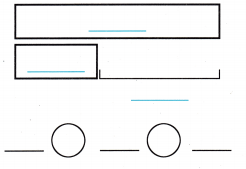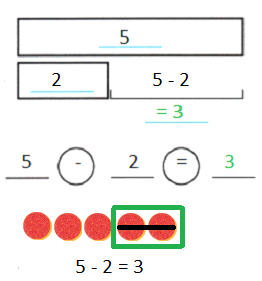Explanation:
Given,
Sally has five feathers,
James has two feathers,
By subtracting two from five we get three,
So Sally has three more feathers than James.

Question 4.
H.O.T. Pam has 4 marbles. Rick has 10 marbles. How many fewer marbles does Pam have than Rick?
________ fewer marbles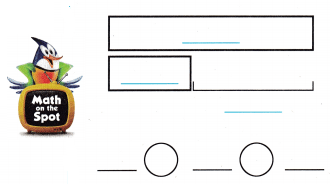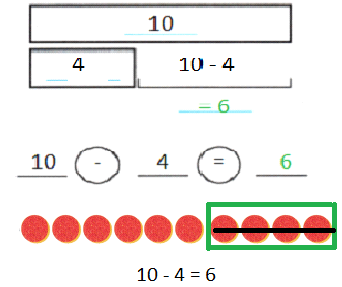Explanation:
Given,
Pam has four marbles,
Rick has ten marbles,
By subtracting four from ten we get six,
So Pam has six fewer marbles than Rick.

Question 5.
Multi-Step Kyle has 6 keys. Kyle has 4 more keys than Lee. How many keys does Lee have?
___________ keys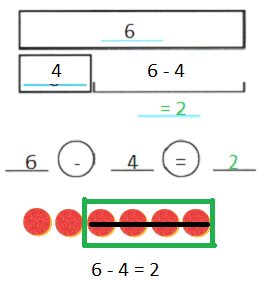Explanation:
Given,
Kyle has six keys,
And Kyle has four more keys than Lee,
By subtracting four from six we get two,
So Lee has two keys.

Use. Choose the correct answer.

Question 6.
Use Diagrams Tony eats 3 treats. Milo eats 5 treats. How many more treats does Milo eat than Tony?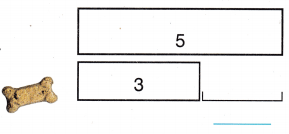(A) 2
(B) 8
(C) 3Explanation:
Given,
Tony eats three treats,
Milo eats five treats,
By subtracting three from five we get two,
So Milo eats two more treats than Tony.

Question 7.
Mondy has 10 beads. Amy has 4 beads. How many fewer beads does Amy have than Mandy?(A) 10
(B) 5
(C) 6Explanation:
Given,
By subtracting four from ten we get six,
So Amy has six fewer beads than Mondy.

Question 8.
Multi-Step Sara finds 9 shells. She finds 4 more shells than Josh. Which number sentence shows how many shells Josh found?(A) 9 – 3 = 6
(B) 11 – 2 = 9
(C) 9 – 4 = 5
Answer: Five ( C ) Option c is right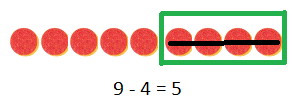Explanation:
Given,
Sara finds nine shells,
Sara finds four more shells than Josh,
By subtracting four from nine we get five,
So Josh found five shells.

Question 9.
Texas Test Prep Sam has three more books than Ed. Sam has 8 books. How many books does Ed have?
(A) 5
(B) 11
(C) 8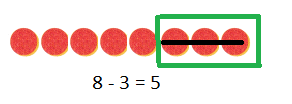Explanation:
Given,
Sam has eight books,
Sam has three more books than Ed,
By subtracting three from eight we get five,
So Ed has five books.

TAKE HOME ACTIVITY • Ask your child to solve this problem. Liz picks 15 flowers, 7 ore pink. The rest are yellow. How many ore yellow?
Answer: there are 8 yellow flowersExplanation:
Given,
Liz picks fifteen flowers,
Seven of them are pink,
By subtracting seven from fifteen we get eight,
So there are eight yellow flowers.

### Texas Go Math Grade 1 Lesson 11.3 Homework and Practice Answer Key

Use the model to solve. Write the number sentence. Then write how many.

Question 1.
9 bees are flying. 4 bees are on flowers. How many more bees are flying than on flowers?_______ more beesGiven:
There are nine bees flying,
Four bees are on flowers,
So by subtracting four from nine we get five,
So there are five more bees flying than on flowers.

Question 2.
Vince has 7 toys. Pat has 4 toys. How many fewer toys does Pat have than Vince?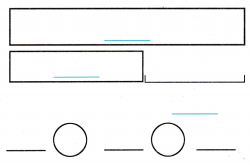________ fewer toys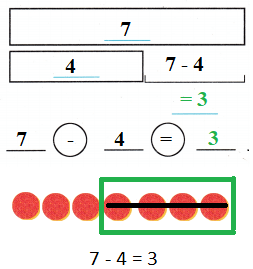Explanation:
Given,
Vince has seven toys,
Pat has four toys,
So by subtracting four from seven we get three,
So Pat has three fewer toys than Vince

Problem Solving

Question 3.
Tim has 8 rulers. Chad has 4 rulers. How many fewer rulers does Chad have than Tim?___________ fewer rulersExplanation:
Given,
Tim has eight rulers,
By subtracting four from eight we get four,
So Chad has four fewer rulers than Tim

Lesson Check

Question 4.
Fido has 12 bones. Champ has 9 bones. How many more bones does Fido have than Champ?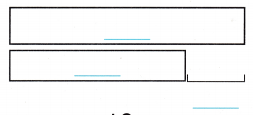(A) 9
(B) 3
(C) 12Explanation:
Given,
Fido has twelve bones,
Champ has nine bones,
By subtracting nine from twelve we get three,
So Fido has three more bones than Champ.

Question 5.
A big squirrel ate 8 nuts. A small squirrel ate 2 nuts. How many fewer nuts did the small squirrel eat?(A) 6
(B) 10
(C) 4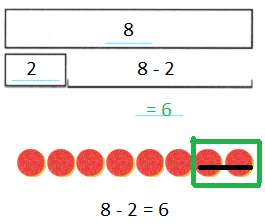Explanation:
Given,
Big squirrel ate eight nuts,
Small squirrel ate two nuts,
By subtracting two from eight we get six,
So a small squirrel has six more nuts to eat than a big squirrel.

Question 6.
Multi-Step Farmer Grey has 10 chicks. He has 3 more chicks than Farmer Brown. Which number sentence shows how many chicks Farmer Brown has?
(A) 10 – 6 = 4
(B) 7 – 3 = 4
(C) 10 – 3 = 7Explanation:
Given,
Farmer Grey has ten chicks,
Farmer Grey has three more chicks more than Farmer Brown,
By subtracting three from ten we get seven,
So Farmer Brown has seven chicks

Question 7.
Lou has 7 marbles. Kim has 2 marbles. How many fewer marbles does Kim have than Lou?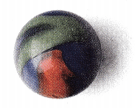(A) 9
(B) 5
(C) 7